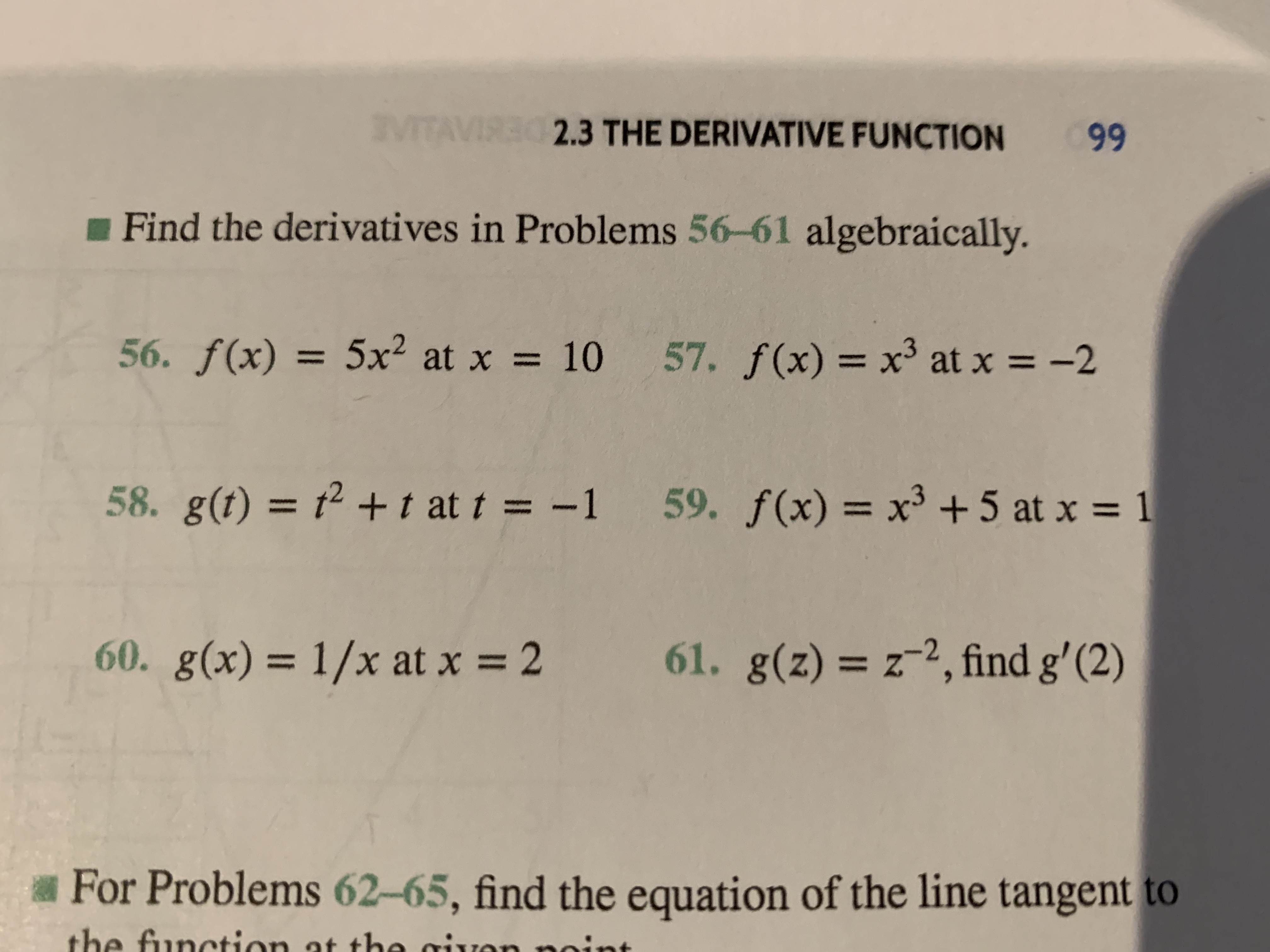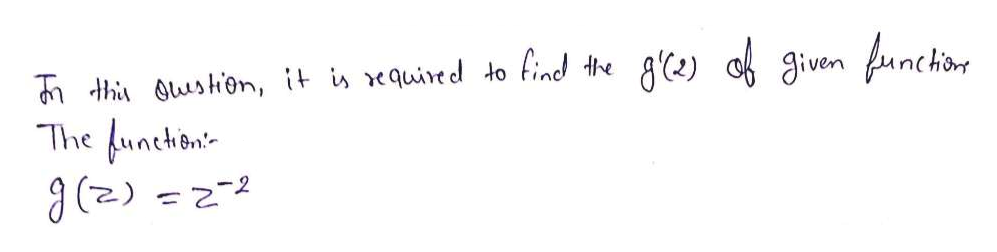VITAVIRE S3 THE DERIVATIVE FUNCTION99Find the derivatives in Problems 56-61 algebraically.56. f(x) = 5x2 at x57. f(x) = x3 at x10-258. g(t) = 2 t at t = -159. f(x) x3 +5 at x161. g(z) z2, find g' (2)60. g(x)=1/x at x=2ZFor Problems 62-65, find the equation of the line tangent tothe function at the givonnin

Questionhelp_outlineImage TranscriptioncloseVITAVIRE S3 THE DERIVATIVE FUNCTION 99 Find the derivatives in Problems 56-61 algebraically. 56. f(x) = 5x2 at x 57. f(x) = x3 at x 10 -2 58. g(t) = 2 t at t = -1 59. f(x) x3 +5 at x 1 61. g(z) z2, find g' (2) 60. g(x)= 1/x at x=2 Z For Problems 62-65, find the equation of the line tangent to the function at the givon nin fullscreen
Step 1help_outlineImage Transcriptioncloseth ushon, it i equired to find the The funchon g'e) cf given unchs 7-2- (2) fullscreen

Want to see the full answer?

See Solution

Want to see this answer and more?

Our solutions are written by experts, many with advanced degrees, and available 24/7

See Solution
Tagged in

Derivative